# Water container

The cube-shaped container is filled to two-thirds of its height. If we pour 18 liters, it will be filled to three-fifths of the height. What is the volume of the whole container?

V =  270 l

### Step-by-step explanation: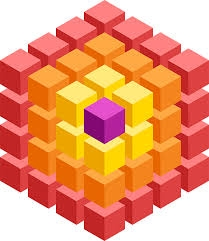Did you find an error or inaccuracy? Feel free to write us. Thank you!Tips to related online calculators
Need help to calculate sum, simplify or multiply fractions? Try our fraction calculator.
Check out our ratio calculator.
Do you have a linear equation or system of equations and looking for its solution? Or do you have a quadratic equation?
Tip: Our volume units converter will help you with the conversion of volume units.

## Related math problems and questions:

• Container with water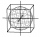The cube-shaped container is filled with water to half its height. If we add 20 liters of water, the container will be filled to three-quarters of its height. What is the volume of the whole container?
• Cube-shaped containerThe cube-shaped container has a height of 52 cm and a square base. The container was filled to the brim with water, then we immersed a metal cube in it, which caused 2.7 l of water to flow out of the container. After removing the cube from the water, the
• Cylinder container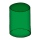If the cylinder-shaped container is filled with water to a height of 5 dm, it contains 62.8 hectoliters of water. Calculate the diameter of the bottom of the container. Use the value π = 3.14.
• Equilateral coneWe pour so much water into a container that has the shape of an equilateral cone, the base of which has a radius r = 6 cm, that one-third of the volume of the cone is filled. How high will the water reach if we turn the cone upside down?
• PebbleThe aquarium with internal dimensions of the bottom 40 cm × 35 cm and a height of 30 cm is filled with two-thirds of water. Calculate how many millimeters the water level in the aquarium rises by dipping a pebble-shaped sphere with a diameter of 18 cm.
• ContainerThe container has a cylindrical shape the base diameter 0.8 m and the area of the base is equal to the area of the wall. How many liters of water can we pour into the container?
• Cylinder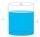The cylinder-shaped container has 80 liters of water. The water reaches 45 cm height. How many water liters will be in a container if the water level extends to a height of 72 cm? Write the result in liters, write down only as a whole or decimal number.
• The hemisphereThe hemisphere container is filled with water. What is the radius of the container when 10 liters of water pour from it when tilted 30 degrees?
• Cylinder-shaped vase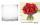If the cylinder-shaped vase is filled with water up to 35 cm, it contains 1 liter of water. How much water will it contain if it is filled to a height of 45cm?
• Water overflow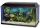A rectangular container that has a length of 30 cm, a width of 20 cm, and a height of 24 cm is filled with water to a depth of 15 cm. When an additional 6.5 liters of water are poured into the container, some water overflows. How many liters of water over
• The cylinder baseThe cylinder with a base of 8 dm2 has a volume of 120 liters. From a cylinder filled with water, 40 liters of water was removed. At what height from the bottom /with precision to dm/ is the water level?
• Water tanksA different amount of water is poured into each of the three identical vessels. In the first vessel, water fills 30% of its volume and in the second vessel 40% of its volume. The third container contains 19 liters of water. If we distributed the water fro
• Mineral water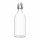The bottle contains 1.5 liters of mineral water. Pour all the water from the bottle into empty cups with a volume of 1/3 l. All but one will be filled to the brim. What part of the volume of the last cup is filled with water?
• Cylinder containerThe cylindrical container with a diameter of 1.8 m contains 2,000 liters of water. How high does the water reach?
• Water level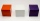How many cm will the water level in a cube-shaped tank with an edge of 3 m drop if we discharge 189 hl of water?
• Wooden containerThe locksmith should cover the cube-shaped wooden container with a metal sheet inside. The outer edge of the container is 54cm. The wall thickness is 25 mm. The container has no lid. Calculate. How many sheets will be needed to cover it?
• Cube containersReplace the two cube-shaped containers with 0.8 dm and 0.6 dm edges with a single cube-shaped one so that it has the same volume as the two original ones together. What is the length of the edge of this cube?